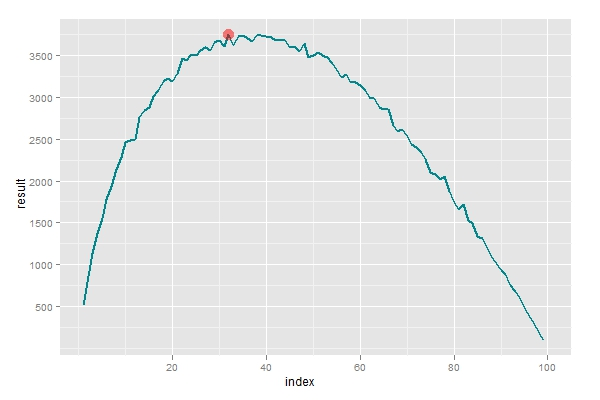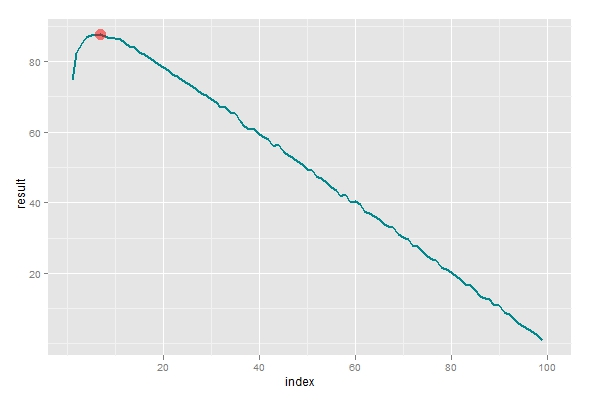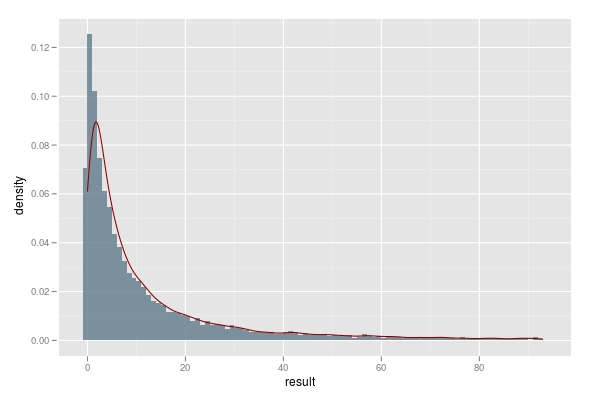谈到相亲就不得不提到著名的麦穗问题。说有一天，苏格拉底带领几个弟子来到一块成熟的麦地边。他对弟子们说：“你们去麦地里摘一个最大的麦穗，但要求只能摘一次，只许进不许退，我在麦地的尽头等你们。”可以看得出，相亲这种活动就有点类似于摘麦穗，在等待和决断之间达成平衡是解决问题的重点。R代码如下：

# 进行观察和选取的函数，n负责对整体进行划分，取值在1到100之间
selection <- function(n) {
raw.data <- sample(1:100,100)
first.group <- raw.data[1:n]
second.group <- raw.data[(n+1):100]
first.max <- max(first.group)
morethan.first <- second.group > first.max
my.select <- ifelse(any(morethan.first) == TRUE,
second.group[morethan.first],0)
return(my.select)
}

# 进行第一次模拟，找到最优的划分参数，使选取到最大值的概率最大。
data <- matrix(rep(0,10000*100),ncol=100)
result1 <- rep(0,100)
for (i in 1:100) {
temp <- replicate(n=10000,selection(i))
data[ ,i] <- temp
result1[i] <- sum(data[,i] == 100)
}
which.max(result1)

# 用ggplot2绘图包进行观察
library(ggplot2)
index <- 1:100
p <- ggplot(data=data.frame(index,result1),aes(index,result1))
p+geom_line(size=1, colour='turquoise4') +
geom_point(aes(x = which.max(result1),y=result1[which.max(result1)]),colour=alpha('red',0.5),size=5)

# 进行第二次模拟，找到最优的划分参数，使选取的期望值达到最大
result2 <- rep(0,100)
for (i in 1:100) {
result2[i] <- mean(replicate(n=10000,selection(i)))
}
p <- ggplot(data=data.frame(index,result2),aes(index,result2))
p+geom_line(size=1, colour='turquoise4') +
geom_point(aes(x = which.max(result2),y=result2[which.max(result2)]),colour=alpha('red',0.5),size=5)

# 构造函数，记录需要多少次尝试才能选取较优的对象
howmany <- function(n) {
raw.data <- sample(1:100,100)
first.group <- raw.data[1:n]
second.group <- raw.data[(n+1):100]
first.max <- max(first.group)
morethan.first <- second.group > first.max
which.select <- ifelse(any(morethan.first) == TRUE,
which(morethan.first==T),0)
return(which.select)
}

# 记录尝试次数的10000次模拟结果，并绘制直方图
result3<- replicate(n=10000,howmany(7))
p <- ggplot(data=data.frame(result3),aes(result3))
p + geom_histogram(binwidth=1, position='identity',
alpha=0.5,fill='lightskyblue4',aes(y = ..density..,))+
stat_density(geom = 'line',colour='red4')

# 观察10次尝试内可选取到合适对象的人数比例
length(result[result>0 & result <10])/10000
length(result[result>0 & result <30])/10000